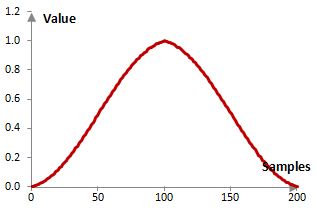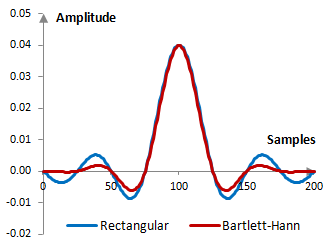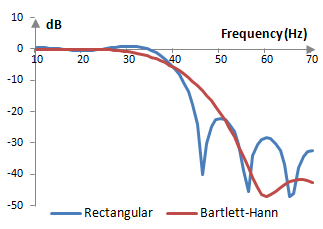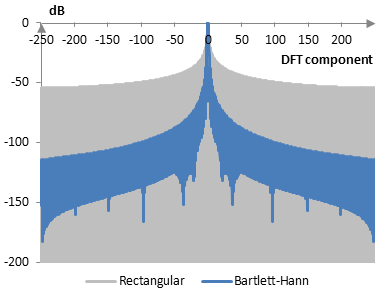# Bartlett-Hann window

The Bartlett-Hann window coefficients are given by the following formula

$$a(k)=0.62-0.48|\frac{k}{N-1}-0.5|-0.38 \, \cos(\frac{2\pi k}{N-1})$$

where N is the length of the filter and k = 0, 1, …, N – 1.

The Bartlett-Hann window is essentially the sum of a Bartlett window (a triangular window) and a Hann window.

## An example Bartlett-Hann window

Consider a finite impulse response (FIR) low pass filter of length N = 201. The following is the Bartlett-Hann window.Given a sampling frequency of 2000 Hz and a filter cutoff frequency of 40 Hz, the impulse response of the filter with a rectangular window (with no window) and with the Bartlett-Hann window is as follows.The magnitude response of the same filter is shown on the graph below.The stop band attenuation of a filter with the Bartlett-Hann window can be as high as -35 dB and as low as -65 dB. The ripples and transition of the Bartlett-Hann window are difficult to quantify, as the filter rarely exhibits ripples. As in the graph above, the magnitude response of the filter tends to show no ripples and to decrease monotonically from the start of the frequency spectrum to the stop band. In the rare cases, where the filter with the Bartlett-Hann window does have ripples, those are usually around +/- 0.02 dB to +/- 0.03 dB. In these cases, the relationship between the length of the filter N and the size of the transition band between 0 dB and the stop band is approximately N = 2.31 / (transition / fs), where fs is the sampling frequency.

## Measures for the Bartlett-Hann window

The following is a comparison of the discrete Fourier transform of the Bartlett-Hann window and the rectangular window.The Bartlett-Hann window measures are as follows.

 Coherent gain 0.5 Equivalent noise bandwidth 1.46 Processing gain -1.64 dB Scalloping loss -1.51 dB Worst case processing loss -3.15 dB Highest sidelobe level -35.9 dB Sidelobe falloff -12.7 dB / octave, -42.3 dB / decade Main lobe is -3 dB 1.40 bins Main lobe is -6 dB 1.94 bins Overlap correlation at 50% overlap 0.186 Amplitude flatness at 50% overlap 1.000 Overlap correlation at 75% overlap 0.674 Amplitude flatness at 75% overlap 1.000

Window

### Filtered HTML

• Freelinking helps you easily create HTML links. Links take the form of [[indicator:target|Title]]. By default (no indicator): Click to view a local node.